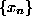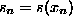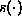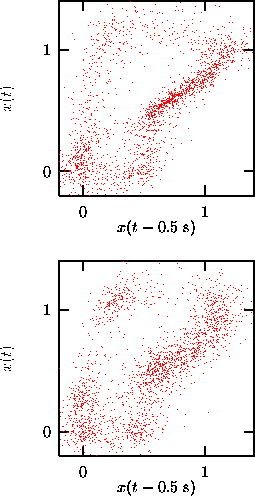Next: General constrained randomization Up: Testing for nonlinearity Previous: The concept of surrogate

## Iterative Fourier transform method

Very few real time series which are suspected to show nonlinearity follow a Gaussian single time distribution. Non-Gaussianity is the simplest kind of nonlinear signature but it may have a trivial reason: The data may have been distorted in the measurement process. Thus a possible null hypothesis would be that there is a stationary Gaussian linear stochastic process that generates a sequence, but the actual observations arewhereis a monotonic function. Constrained realizations of this null hypothesis would require the generation of random sequences with the same power spectrum (fully specifying the linear process) and the same single time distribution (specifying the effect of the measurement function) as the observed data. The Amplitude Adjusted Fourier Transform (AAFT) method proposed in  attempts to invert the measurement functionby rescaling the data to a Gaussian distribution. Then the Fourier phases are randomized and the rescaling is inverted. As discussed in , this procedure is biased towards a flatter spectrum since the inverse ofis not available exactly. In the same reference, a scheme is introduced that removes this bias by iteratively adjusting the spectrum and the distribution of the surrogates. Alternatingly, the surrogates are rescaled to the exact values taken by the data and then the Fourier transform is brought to the exact amplitudes obtained from the data. The discrepancy between both steps either converges to zero with the number of iterations or to a finite inaccuracy which decreases with the length of the time series. The program surrogates performs iterations until no further improvement can be made. The last two stages are returned, one having the exact Fourier amplitudes and one taking on the same values as the data. For not too exotic data these two versions should be almost identical. The relative discrepancy is also printed.Figure:   Upper: The human breath rate data from Fig.. Lower: the noise component extracted by the noise reduction scheme has been randomized in order to destroy correlations with the signal. The result appears slightly but significantly less structured than the original.

In Fig.we used this procedure to assess the hypothesis that the noise reduction on the breath data reported in Fig.removed an additive noise component which was independent of the signal. If the hypothesis were true, we could equally well add back on the noise sequence or a randomized version of it which lacks any correlations to the signal. In the upper panel of Fig.we show the original data. In the lower panel we took the noise reduced version (c.f. Fig., bottom) and added a surrogate of the supposed noise sequence. The result is similar but still significantly different from the original to make the additivity assumption implausible.

Fourier based randomization schemes suffer from some caveats due to the the inherent assumption that the data constitutes one period of a periodic signal, which is not what we really expect. The possible artefacts are discussed for example in  and can, in summary, lead to spurious rejection of the null hypothesis. One precaution that should be taken when using surrogates is to make sure that the beginning and the end of the data approximately match in value and phase. Then, the periodicity assumption is not too far wrong and harmless. Usually, this amounts to the loss of a few points of the series. One should note, however, that the routine may truncate the data by a few points itself in order to be able to perform a fast Fourier transform which requires the number of points to be factorizable by small prime factors.Next: General constrained randomization Up: Testing for nonlinearity Previous: The concept of surrogate

Thomas Schreiber
Wed Jan 6 15:38:27 CET 1999